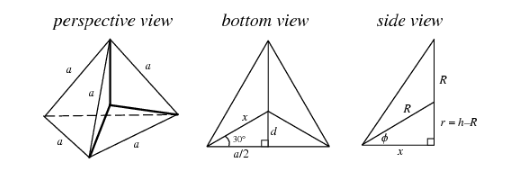The inradius of a regular tetrahedron of side length a isA. $\sqrt {\dfrac{2}{3}a}$ B. $\sqrt {\dfrac{3}{2}a}$ C. $\dfrac{a}{{\sqrt 6 }}$ D. $\dfrac{a}{{2\sqrt 6 }}$Verified
120.3k+ views
Hint: In the problem they have asked to find the inradius of a regular tetrahedron. Let the side length of a tetrahedron be $a$ , and let its base lie in the plane $z = 0$ with one vertex lying along the positive $x$ axis. Thereafter, assuming the inradius and the circumference to be $r$ and $R$ respectively.ording to the given information, we have,
Side length of a tetrahedron is $a$ .
Let its base lie in the plane $z = 0$ with one vertex lying along the positive $x$ axis. Assuming the inradius and the circumference to be $r$ and $R$ respectively and also the height and area to be $h$ and $A$ respectively.
Then the polyhedron vertices of this tetrahedron are located at ( $x,0,0$ ), ( $- d, \pm \dfrac{a}{2},0$ ), and ( $0,0,h$ ), where,
$\Rightarrow x = \dfrac{{\dfrac{a}{2}}}{{\cos (\dfrac{\pi }{6})}}$ $= \dfrac{1}{3}\sqrt {3}a$
Thereafter on simplifying ‘ $d$ ’ we get,
$\Rightarrow d = \sqrt {{x^2} - {{(\dfrac{1}{2}a)}^2}}$ $= \dfrac{1}{6}\sqrt {3}a$
This further gives the area of the base as,
$\Rightarrow A = \dfrac{1}{2}a(R + x)$ $= \dfrac{1}{4}\sqrt 3 {a^2}$
Therefore we get the height of the tetrahedron to be,
$\Rightarrow h = \sqrt {{a^2} - {x^2}}$ $= \dfrac{1}{3}\sqrt 6 a$
Further, the circumference of the tetrahedron is calculated from,
${x^2} + {(h - R)^2} = {R^2}$
${x^2} + {h^2} - 2hR + {R^2} = {R^2}$
Now on substituting the values already calculated in the above given equation we get,
$\Rightarrow R = \dfrac{{{x^2} + {h^2}}}{{2h}}$
$\Rightarrow \dfrac{1}{4}\sqrt 6 a$
Finally, we calculate the inradius $r$ from,
$r = h - R$
$\Rightarrow \dfrac{1}{{12}}\sqrt 6 a$ $= \dfrac{a}{{2\sqrt 6 }}$
So, the correct answer is “Option D”.

Note: Tetrahedron is the simplest of the entire ordinary convex polygon and the only one which has fewer than five faces (four faces). The tetrahedron has 7 axes of symmetry: $^4{C_3}$ (axes connecting vertices with the centers of the opposite faces) and $^3{C_2}$ (the axes connecting the midpoints of opposite sides).